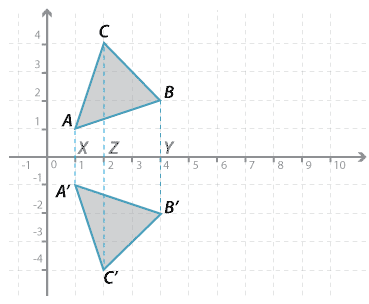### Reflection in the x-axisDetailed description

The point $$A$$ in the diagram above has been reflected in the $$x$$-axis. To reflect $$A$$, a line has been drawn at right angles to the axis of reflection the (the $$x$$-axis in this case) and a ruler or compass has been used to mark $$A^\prime$$ the same distance from the axis of reflection as $$A$$, but on the other side. The same has been done for $$B$$ and $$C$$.

Using coordinates we can describe the reflection of the vertices:

$$A(1, 1) \rightarrow A^\prime (1, −1); B(4, 2) \rightarrow B^\prime (4, −2); C(2, 4) \rightarrow C^\prime (2, −4)$$